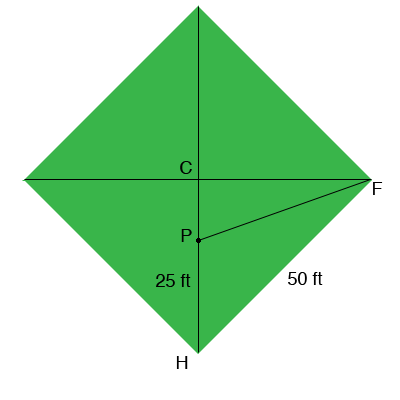SEARCH HOMEMath Central Quandaries & QueriesQuestion from Svitlana, a student: The Adam's family has set up a wiffleball field in their backyard. The bases are arranged like a typical baseball diamond, where the distance between consecutive bases is the same. First base is opposite of third base, and second base is opposite of home plate. The distance between consecutive bases is 50 feet. Now, the pitcher stands 25 feet from home plate and lies on the line between home plate and second base. How far is the pitcher from first base? Round your answer down to the nearest inch.Hi Svitlana,

I drew the wiffleball field you described and labeled some points.Triangle HFC is an isosceles right angle triangle so you can use Pythagoras Theorem to find the length of CH. The pitcher is at P, 25 feet from home plate H and hence you now know the distance from C to P. Now use Pythagoras Theorem on the triangle FCP to fond the distance from P to first base F.

PennyMath Central is supported by the University of Regina and The Pacific Institute for the Mathematical Sciences.